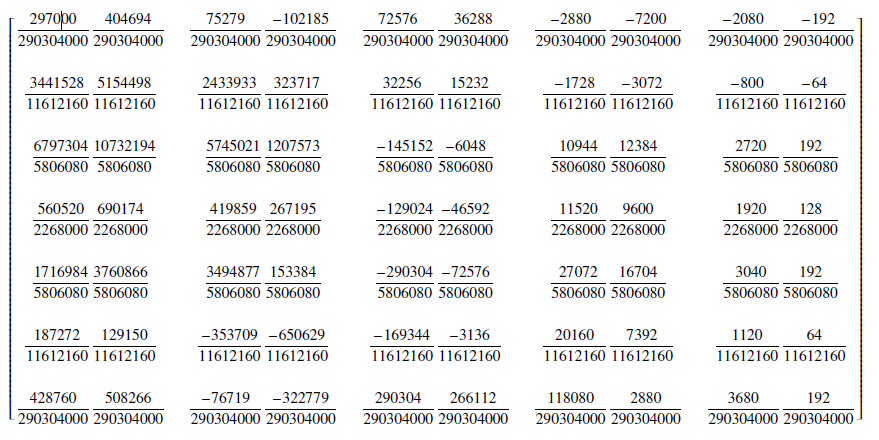# Numerical Algorithms for Direct Solution of Fourth Order Ordinary Differential Equations

## Authors

• J. O. Kuboye Department of Mathematics, Federal University Oye-Ekiti, Oye-Ekiti, Nigeria
• O. R. Elusakin Department of Mathematics, Federal University Oye-Ekiti, Oye-Ekiti, Nigeria
• O. F. Quadri Department of Mathematics, Federal University Oye-Ekiti, Oye-Ekiti, Nigeria

## Keywords:

Interpolation, Collocation, Block methods, Fourth order, Ordinary differential equations

## Abstract

This paper examines the derivation of hybrid numerical algorithms with step length(k) of five for solving fourth order initial value problems of ordinary differential equations directly. In developing the methods, interpolation and collocation techniques are considered. Approximated power series is used as interpolating polynomial and its fourth derivative as the collocating equation. These equations are solved using Gaussian-elimination approach in finding the unknown variables aj, j=0,...,10 which are substituted into basis function to give continuous implicit scheme. The discrete schemes and its derivatives that form the block are obtained
by evaluating continuous implicit scheme at non-interpolating points. The developed methods are of order seven and the results generated when the methods were applied to fourth order initial value problems compared favourably with existing methods.order initial value problems compared favourably with existing methods.

Dimensions

D. A. Awoyemi, “Class of continuous methods for general second order initial value problems in ordinary differential equations”, International Journal of Computer Mathematics 72 (2009) 29.

S. O. Fatunla, Numerical methods for initial value problems in ordinary differential equations, Academic press Inc. Harcourt Brace Jovanovich Publishers, New York. (1988) DOI: https://doi.org/10.1016/B978-0-12-249930-2.50012-6

J. D. Lambert, Computational method in ordinary differential equation, John Wiley and Sons, Inc. (1973)

O. C. Akeremale, J. O. Kuboye, S. H. Yeak, E. A. Abununyi & S. Olaiju, ”Hybrid-block numerical method for solving second order ordinary differential equations”, International Journal of Computational Analysis 3 (2020) 25.

O. E. Abolarin, E. O. Adeyefa, J. O. Kuboye & B. G. Ogunware, “A novel multi derivative hybrid method for numerical treatment of higher order ordinary differential equations”, Al Dar Research Journal of Sustainability 4 (2020) 43.

J. O. Kuboye, Z. Omar, O. E. Abolarin & R. Abdelrahim, “Generalized hybrid block method for solving second order ordinary differential equations directly”, Research and Reports on Mathematics 2 (2018) 2.

Z. Omar & J. O. Kuboye, “Comparison of block methods with different step-lengths for solving second order ordinary differential equations”, Journal of Computational and Theoretical Nanoscience Science and Technology

(2018) 966.

E.O. Adeyefa, “Orthogonal-based hybrid block method for solving general second order initial value problems,” Italian Journal of Pure and Applied Mathematics 37 (2017) 659.

Y. A. Abdullahi, Z. Omar & J. O. Kuboye, “Derivation of block predictor–block corrector method for direct solution of third order ordinary differential equations”, Global Journal of Pure and Applied Mathematics 12 (2016) 342.

U. Mohammed & R.B. Adeniyi, “Derivation of five –step block hybrid backward differential formulas (HBDF) through the continuous multistep collocation for solving second order differential equation”, Pacific Journal of Science and Technology 15 (2014) 89.

B. T. Olabode, “An accurate scheme by block method for the third order ordinary differential equation”, Pacific Journal of Science and Technology10 (2009) 136.

A. O. Adesanya, M. O. Udoh & A. M. Ajileye, “A new hybrid block method for direct solution of general third order initial value problems of ordinary differential equations”, International Journal of Pure and Applied Mathematics 4 (2013) 42.

Z. Omar & M. Suleiman, “Solving higher order odes directly using parallel 2-point explicit block method”, Matematika. Pengintegrasian Teknologi Dalam Sains Matematik. Universiti Sains Malaysia 21 (2005) 15.

A. B. Familua & E. O. Omole, “Five points mono hybrid linear multistep method for solving nth order ordinary differential equations using power series function”, Asian Research Journal of Mathematics (Science Domain International) 3 (2017) 1.

J. O. Kuboye & Z. Omar, “New Zero-stable block method for direct solution of fourth order ordinary differential equation”, Indian Journal of Science and Technology 8 (2015) 1.

E. A. Areo & E. O. Omole, “Half-Step symmetric continuous hybrids block method for the numerical solutions of fourth order ordinary differential equations”, Archives of Applied Science Research 7 (2015) 39.

U. Mohammed, “A six step block method for solution of fourth order ordinary differential equations”, The Pacific Journal of Science and Technology 11 (2010) 259.2020-11-15

## How to Cite

Kuboye, J., Elusakin, O. R., & Quadri, O. F. (2020). Numerical Algorithms for Direct Solution of Fourth Order Ordinary Differential Equations. Journal of the Nigerian Society of Physical Sciences, 2(4), 218–227. https://doi.org/10.46481/jnsps.2020.100

## Section

Original Research